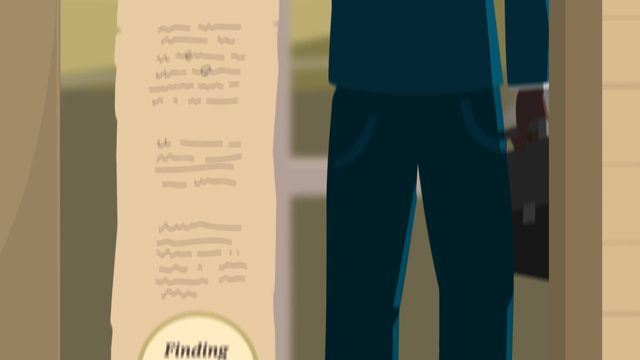# The Area of a CircleRate this video

Ø 5.0 / 1 ratings

The authorTrentkay

## Information about the videoThe Area of a Circle

After this lesson you will be able to derive and use the formula for the area of a circle.

The lesson begins with dividing the circle into equal pieces and rearranging them into a shape that resembles a rectangle. It leads to using the area of a rectangle approximation to discover the area of a circle. It concludes with using the area formula to calculate the area of circles.

Learn about the formula for the area of circles by helping Farmer Jack recover from his devastating loss!

END PREVIEW

This video includes key concepts, notation, and vocabulary such as: radius (a line from a circle’s center to edge), diameter (a line passing through a circle’s center, connecting two points on the edge), circumference (the distance around a circle), and pi (π) (the ratio between a circle’s circumference and diameter).

Before watching this video, you should already be familiar with the area of a rectangle, and translating words into mathematical expressions/equations.

After watching this video, you will be prepared to learn how to determine the area of partial circle regions and composite shapes.

Common Core Standard(s) in focus: 7.G.B.4, 7.G.B.6 A video intended for math students in the 7th grade Recommended for students who are 12-13 years